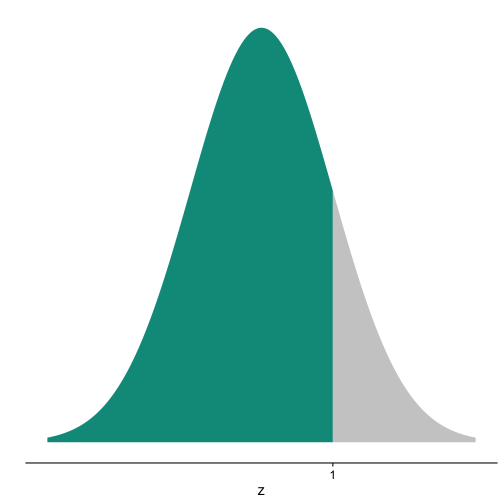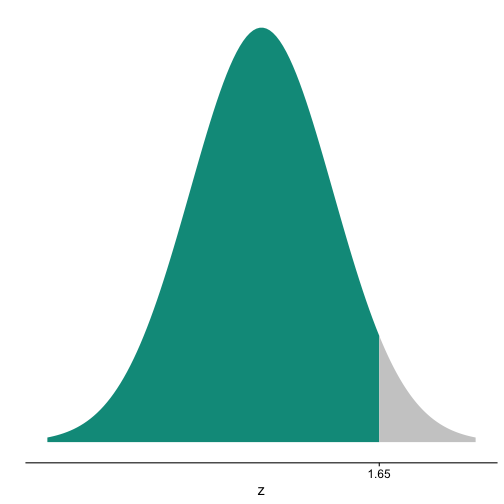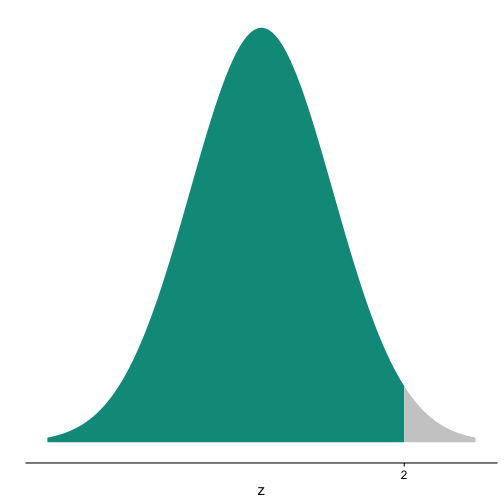# Shading normal curve made easy

## August 29, 2017

Reading time ~2 minutes

Shading values/areas under the normal curve is a quite frequent taks in eg educational contexts. Thanks to Hadley in this post, I found this easy solution.

library(ggplot2)


r
ggplot(NULL, aes(c(-3,3))) +
geom_area(stat = "function", fun = dnorm, fill = "#00998a", xlim = c(-3, 0)) +
geom_area(stat = "function", fun = dnorm, fill = "grey80", xlim = c(0, 3))Simple, right?

Some minor beautification:

 ggplot(NULL, aes(c(-3,3))) +
geom_area(stat = "function", fun = dnorm, fill = "#00998a", xlim = c(-3, 1)) +
geom_area(stat = "function", fun = dnorm, fill = "grey80", xlim = c(1, 3)) +
labs(x = "z", y = "") +
scale_y_continuous(breaks = NULL) +
scale_x_continuous(breaks = 1)And some other quantiles:

ggplot(NULL, aes(c(-3,3))) +
geom_area(stat = "function", fun = dnorm, fill = "#00998a", xlim = c(-3, 1.65)) +
geom_area(stat = "function", fun = dnorm, fill = "grey80", xlim = c(1.65, 3)) +
labs(x = "z", y = "") +
scale_y_continuous(breaks = NULL) +
scale_x_continuous(breaks = 1.65)ggplot(NULL, aes(c(-3,3))) +
geom_area(stat = "function", fun = dnorm, fill = "#00998a", xlim = c(-3, 2)) +
geom_area(stat = "function", fun = dnorm, fill = "grey80", xlim = c(2, 3)) +
labs(x = "z", y = "") +
scale_y_continuous(breaks = NULL) +
scale_x_continuous(breaks = 2)
`### This blog has moved

This blog has moved to Adios, Jekyll. Hello, Blogdown!… Continue reading

#### Wie gut schätzt eine Stichprobe die Grundgesamtheit?

Published on November 17, 2017

#### Some thoughts on tidyveal and environments in R

Published on November 16, 2017